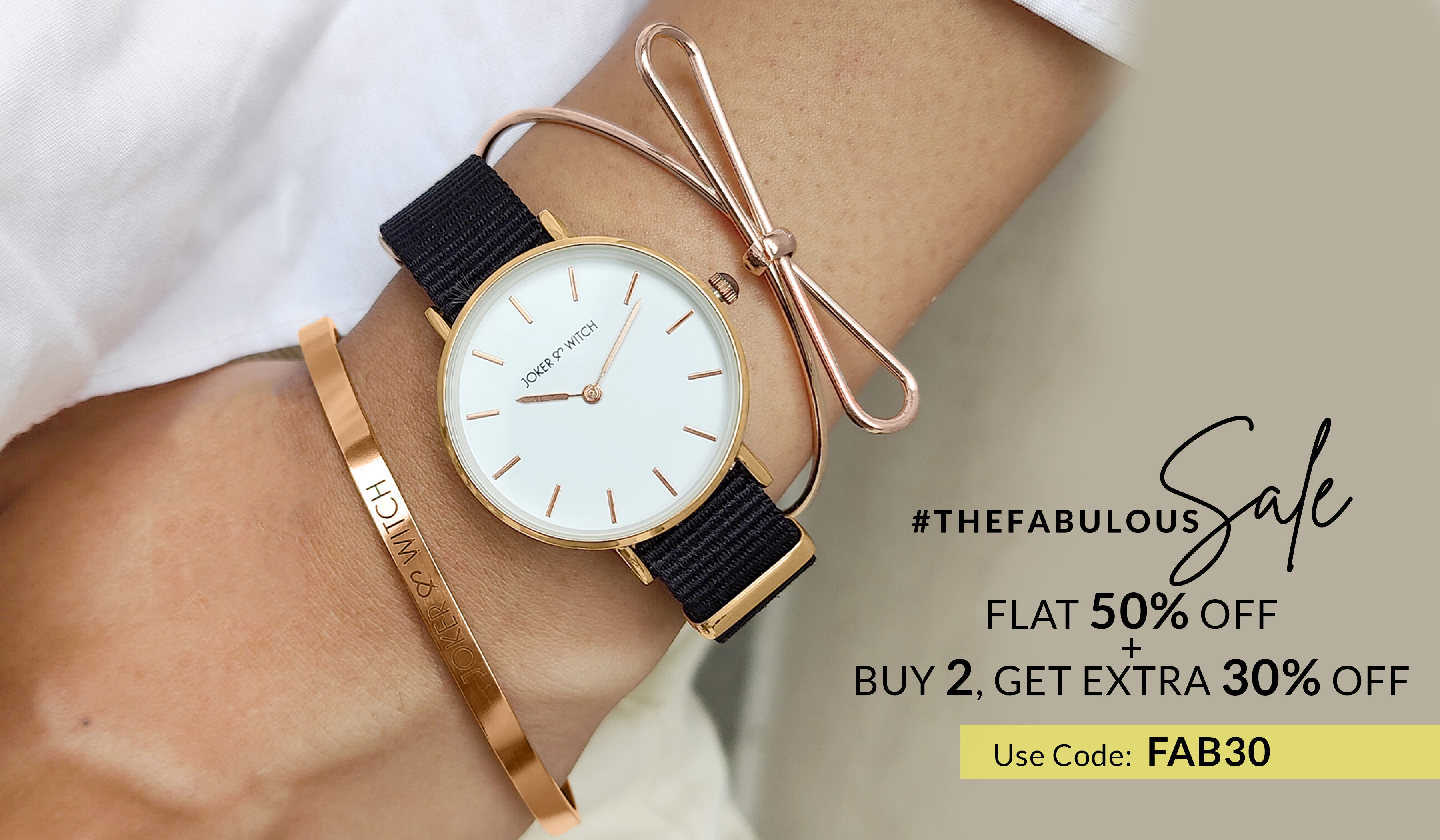# Bracelet Stacks• Sort by

• Shop by Color

• Shop by Style

• Shop by Type

SALE

Rs. 3,999.00
Rs. 5,599.00
SOLD OUT

Rs. 2,699.00
Rs. 4,999.00
SALE

Rs. 3,499.00
Rs. 6,199.00
SOLD OUT

Rs. 2,299.00
Rs. 4,599.00
SOLD OUT

Rs. 2,499.00
Rs. 4,999.00
SOLD OUT

Rs. 2,299.00
Rs. 4,599.00
SALE

Rs. 2,599.00
Rs. 5,199.00
SOLD OUT

Rs. 2,599.00
Rs. 5,199.00
SALE

Rs. 2,599.00
Rs. 5,199.00
SALE

Rs. 2,299.00
Rs. 4,599.00
SALE

Rs. 2,899.00
Rs. 5,799.00
SOLD OUT

Rs. 2,899.00
Rs. 5,799.00
SOLD OUT

Rs. 2,499.00
Rs. 4,999.00
SOLD OUT

Rs. 2,399.00
Rs. 4,799.00
SOLD OUT

Rs. 2,799.00
Rs. 5,599.00
SALE

Rs. 2,799.00
Rs. 5,599.00
SOLD OUT

Rs. 2,799.00
Rs. 5,599.00
SOLD OUT

Rs. 2,499.00
Rs. 4,999.00
SALE

Rs. 2,799.00
Rs. 5,699.00
SALE

Rs. 2,799.00
Rs. 5,599.00
SOLD OUT

Rs. 2,699.00
Rs. 5,399.00
SALE

Rs. 2,299.00
Rs. 4,599.00
SOLD OUT

Rs. 2,899.00
Rs. 5,899.00
SOLD OUT

Rs. 2,899.00
Rs. 5,799.00
SALE

Rs. 2,250.00
Rs. 4,499.00
SOLD OUT

Rs. 2,350.00
Rs. 4,699.00
SALE

Rs. 2,100.00
Rs. 4,199.00
SOLD OUT

Rs. 2,250.00
Rs. 4,499.00
SOLD OUT

Rs. 2,150.00
Rs. 4,299.00
SALE

Rs. 2,250.00
Rs. 4,499.00
SOLD OUT

Rs. 1,800.00
Rs. 3,599.00
SALE

Rs. 2,450.00
Rs. 4,899.00
SALE

Rs. 2,350.00
Rs. 4,699.00
SALE

Rs. 2,350.00
Rs. 4,699.00
SOLD OUT

Rs. 2,450.00
Rs. 4,899.00
SALE

Rs. 2,550.00
Rs. 5,099.00
SALE

Rs. 2,250.00
Rs. 4,499.00
SALE

Rs. 2,350.00
Rs. 4,699.00
SALE

Rs. 2,550.00
Rs. 5,099.00
SALE

Rs. 2,450.00
Rs. 4,899.00
SALE

Rs. 2,550.00
Rs. 5,099.00
SOLD OUT

Rs. 2,450.00
Rs. 4,899.00
SOLD OUT

Rs. 2,150.00
Rs. 4,299.00
SALE

Rs. 2,150.00
Rs. 4,299.00
SALE

Rs. 2,100.00
Rs. 4,199.00
SALE

Rs. 2,200.00
Rs. 4,399.00
SALE

Rs. 2,100.00
Rs. 4,199.00
SOLD OUT

Rs. 2,200.00
Rs. 4,399.00
Back to the top